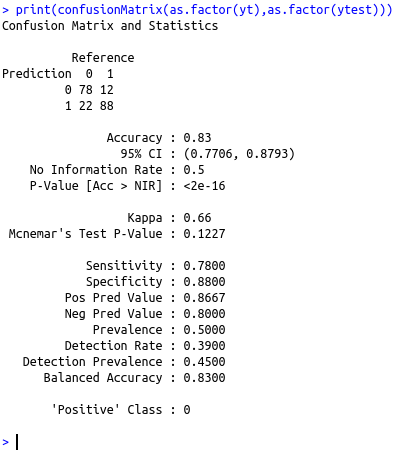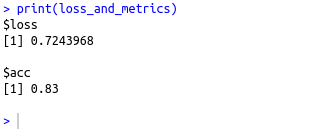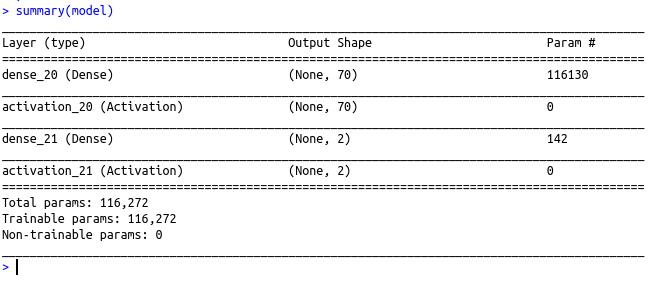• #5, First Floor, 4th Street Dr. Subbarayan Nagar Kodambakkam, Chennai-600 024 Landmark : Samiyar Madam
• pro@slogix.in
• +91- 81240 01111

How to perform sentiment analysis for the amazon review data set using deep neural network in R?

Description

To perform sentiment analysis for the amazon review data set using deep neural network in R

Functions Used

model layer_dense() – Add a input,output or hidden layer
layer_activation() – Activation function for the layer
compile() – To compile the model
fit() – To fit the model using the train set
predict() – To predict using the test set
evaluate() – To evaluate the loss and metrics

Libraries Required :

library(keras)

Process

Convert the categorical variables to equivalent numeric classes

Split the data set as train set and test set

Initialize the keras sequential model

Build the model with input layers,hidden layers and output layer as per the data size along with the activation function(here 1658(vectorizer count) I/P layer with relu activation and 2 O/P layer with sigmoid activation)

Compile the model with required loss,metrics and optimizer(here loss=binary_crossentropy,optimizer=adam,metrics=accuracy)

Fit the model using the train set

Predict using the test set

Evaluate the metrics

Sapmle Code

library(“caret”)
library(“text2vec”)
library(keras)
data data1=(strsplit(data\$text,”\n”))
data2=unlist(data1[])
data3=strsplit(data2,”\t”)
data4=unlist(data3)
i=0
j=0
k=0
text=c()
pol=c()
for (i in (1:length(data4)))
{
if(i%%2!=0)
{
j=j+1
text[j]=data4[i]
}else
{
k=k+1
pol[k]=data4[i]
}
}
df=data.frame(text=text,polarity=pol,stringsAsFactors = FALSE)
#Setting seed to reproduce results of random sampling data
set.seed(100)
#Train set
train_ind<-createDataPartition(df\$polarity,p=0.8,list=FALSE)
train_data<-df[train_ind,]
xtrain=train_data\$text
ytrain=train_data\$polarity
#Test set
test=df[-train_ind,]
xtest=test\$text
ytest=as.numeric(test[[‘polarity’]])
#Define preprocessing function and tokenization function
prep_fun = tolower
tok_fun = word_tokenizer
#create vector of tokens and build the vocabulary
it_train = itoken(train_data\$text,
preprocessor = prep_fun,
tokenizer = tok_fun,
progressbar = FALSE)
vocab = create_vocabulary(it_train)
vectorizer = vocab_vectorizer(vocab)
#Create the document term matrix(DTM) for train set
dtm_train = create_dtm(it_train, vectorizer)
#Create vector of tokens for test set
it_test = itoken(xtest,
preprocessor = prep_fun,
tokenizer = tok_fun,
progressbar = FALSE)
#Create the document term matrix(DTM) for test set
dtm_test=create_dtm(it_test,vectorizer)
ytrain=factor(ytrain)
ytest=factor(ytest)
#converting the target variable to once hot encoded vectors using keras inbuilt function
train_y<-to_categorical(ytrain)
test_y #defining a keras sequential model
model %
layer_dense(units = 70, input_shape = 1658 )%>%
layer_activation(activation = ‘relu’) %>%
layer_dense(units = 2)%>%
layer_activation(activation= “sigmoid”)
#compiling the defined model with metric = accuracy and optimiser as adam.
model %>% compile(
loss = ‘binary_crossentropy’,
metrics = ‘accuracy’
)
#Summary of the model
summary(model)
#fitting the model on the training dataset
model %>%keras:: fit(dtm_train, train_y, epochs = 200,batch_size=20)
#Predict using the Test data
yt=predict_classes(model,dtm_test)
loss_and_metrics % evaluate(dtm_test, test_y)
print(loss_and_metrics)
cat(“The confusion matrix is \n”)
print(confusionMatrix(as.factor(yt),as.factor(ytest)))

Screenshots